Picture by Design Cue, Unsplash.

Pearson’s product-moment correlation, or Pearson’s r, is a statistical method commonly used in data science to measure the strength of the linear relationship between variables. If you can identify existing features, or engineer new ones, that either have a strong correlation with your target variable, you can help improve your model’s performance.

The Pearson correlation coefficient examines two variables, X and y, and returns a value between -1 and 1, indicating the strength of their linear correlation. A value of -1 is a perfect negative correlation, a value of exactly 0 indicates no correlation, while a value of 1 indicates a perfect positive correlation.

Since Pearson’s R shows a linear relationship, you can visualise the relationships between variables using scatter plots with regression lines fitted. A regression line that slopes upwards to the right indicates a strong positive correlation, a regression line that slopes downwards to the left indicates a strong negative correlation, while a flat line indicates no correlation.

Let’s take a look at some simple ways you can measure the correlation between variables within your data set, and examine their specific relationships to the target variable your model is aiming to predict.

For this project we’ll be using Pandas and Numpy for loading and manipulating data, and Matplotlib and Seaborn for creating visualisations to help us identify correlations between the variables.

``````import pandas as pd
import numpy as np
import matplotlib.pyplot as plt
import seaborn as sns
``````

You can use any data you like, but I’m using the California Housing data set. You can download this directly from my GitHub using the Pandas `read_csv()` function and then display the data in a transposed Pandas dataframe using `df.head().T`.

``````df = pd.read_csv('https://raw.githubusercontent.com/flyandlure/datasets/master/housing.csv')
``````
0 1 2 3 4
longitude -122.23 -122.22 -122.24 -122.25 -122.25
latitude 37.88 37.86 37.85 37.85 37.85
housing_median_age 41 21 52 52 52
total_rooms 880 7099 1467 1274 1627
total_bedrooms 129 1106 190 235 280
population 322 2401 496 558 565
households 126 1138 177 219 259
median_income 8.3252 8.3014 7.2574 5.6431 3.8462
median_house_value 452600 358500 352100 341300 342200
ocean_proximity NEAR BAY NEAR BAY NEAR BAY NEAR BAY NEAR BAY

At the moment, some of the most useful features are currently categorical variables. To examine their correlation to the target variable `median_house_price`, these need to be transformed into numeric variables. To do this we’ll use the one-hot encoding technique via the Pandas `get_dummies()` function. Converting the column values to lowercase and slugifying them keeps the column names created a bit neater.

``````df['ocean_proximity'] = df['ocean_proximity'].str.lower().replace('[^0-9a-zA-Z]+','_',regex=True)
encodings = pd.get_dummies(df['ocean_proximity'], prefix='proximity')
df = pd.concat([df, encodings], axis=1)
df.sample(5).T
``````
2517 15071 8775 12352 17142
longitude -122.16 -116.98 -118.31 -116.54 -122.18
latitude 39.78 32.79 33.8 33.81 37.45
housing_median_age 32 32 29 31 43
total_rooms 1288 3756 2795 6814 2061
total_bedrooms 221 662 572 1714 437
population 562 1611 1469 2628 817
households 203 598 557 1341 385
median_income 2.325 3.8667 3.7167 2.1176 4.4688
median_house_value 69600 189700 308900 124100 460200
ocean_proximity inland _1h_ocean _1h_ocean inland near_bay
proximity__1h_ocean 0 1 1 0 0
proximity_inland 1 0 0 1 0
proximity_island 0 0 0 0 0
proximity_near_bay 0 0 0 0 1
proximity_near_ocean 0 0 0 0 0

### Calculate correlation to the target variable

The first way to calculate and examine correlations is to do it via Pandas. This comes with a function called `corr()` which calculates the Pearson correlation. If you provide the name of the target variable column `median_house_value` and then sort the values in descending order, Pandas will show you the features in order of correlation with the target.

At the top we have a very strong positive correlation with `median_income` - the higher this value, the higher the value of the house. At the bottom we have a strong negative correlation with `proximity_inland` - the further inland, the lower the house value. The values that are close to zero may not add a great deal individually, but often contribute when combined with other variables.

``````df[df.columns[1:]].corr()['median_house_value'][:].sort_values(ascending=False).to_frame()
``````
median_house_value
median_house_value 1.000000
median_income 0.688075
proximity__1h_ocean 0.256617
proximity_near_bay 0.160284
proximity_near_ocean 0.141862
total_rooms 0.134153
housing_median_age 0.105623
households 0.065843
total_bedrooms 0.049686
proximity_island 0.023416
population -0.024650
latitude -0.144160
proximity_inland -0.484859

### Create a correlation heatmap

To visualise the correlations between all variables, not just the target variable, you can create a correlation matrix. This is essentially the same as the dataframe above, but with a row for each variable, and a neat colour coding scheme that allows you to see which values are most positively or negatively correlated based on the depth of their colour. Pale cells denote values with a negative correlation, while dark cells denote a stronger positive correlation. The `fmt='.1g'` argument reduces the number of decimal points, where it’s feasible to do so, to aid readability.

``````plt.figure(figsize=(14,8))
sns.set_theme(style="white")
corr = df.corr()
heatmap = sns.heatmap(corr, annot=True, cmap="Blues", fmt='.1g')
``````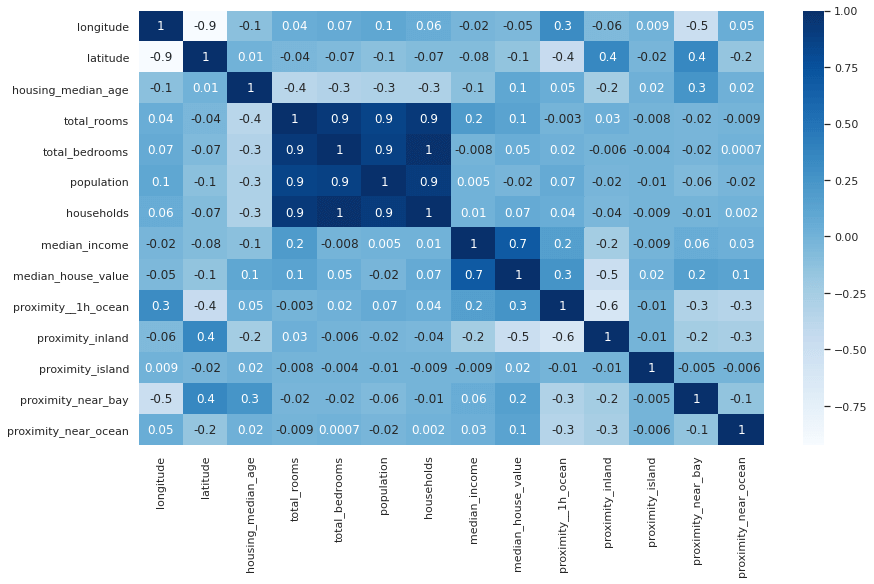If you find it easier to read without the annotations showing the Pearson correlation score, you can remove the `annot=True` argument from the Seaborn `heatmap()` function and get a more minimalist plot. You can also change the colour map by using a different value in the `cmap` argument.

``````plt.figure(figsize=(14,8))
sns.set_theme(style="white")
corr = df.corr()
heatmap = sns.heatmap(corr, cmap="coolwarm")
``````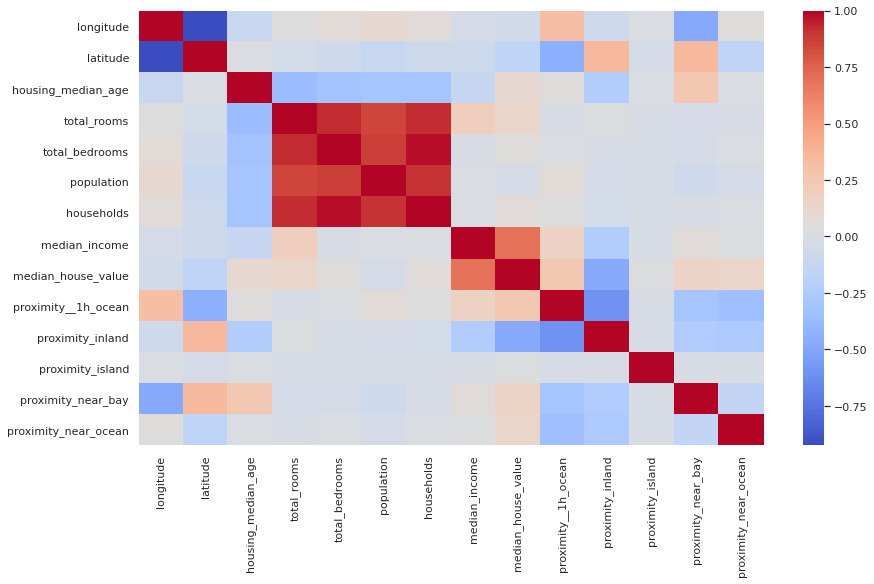### Diagonal correlation matrix

If you look closely at the correlation matrix above, you’ll notice that the data are repeated either side of the diagonal row. To get rid of the diagonal row, which shows the correlation of the variable with itself, and is therefore always 1, you can use a mask technique and some funky Numpy code to blank the cells out. To my eye, the diagonal correlation matrix is much easier to read.

``````sns.set_theme(style="white")
corr = df.corr()
f, ax = plt.subplots(figsize=(10, 10))
cmap = sns.color_palette("Blues")

sns.heatmap(corr,
cmap=cmap,
vmax=.3,
center=0,
square=True,
linewidths=3,
cbar_kws={"shrink": .5}
)
``````
``````<matplotlib.axes._subplots.AxesSubplot at 0x7f749ffc02e0>
``````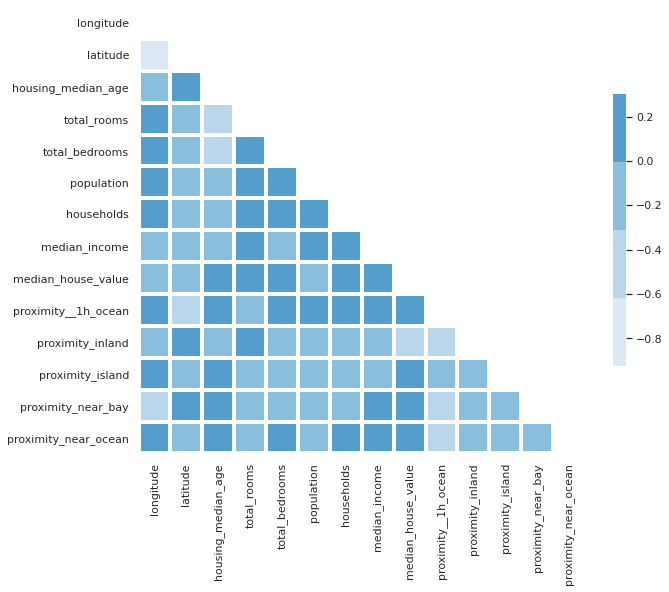### Pairplots

Pairplots are also a useful to examine the relationships between data. The snag with these, however, is that they produce truly massive plots on larger datasets that can take some time to generate. Adding the `kind="reg"` argument adds a regression line to make spotting trends a bit easier.

``````sns.pairplot(df, kind="reg")
``````
``````<seaborn.axisgrid.PairGrid at 0x7f749ffbeb20>
``````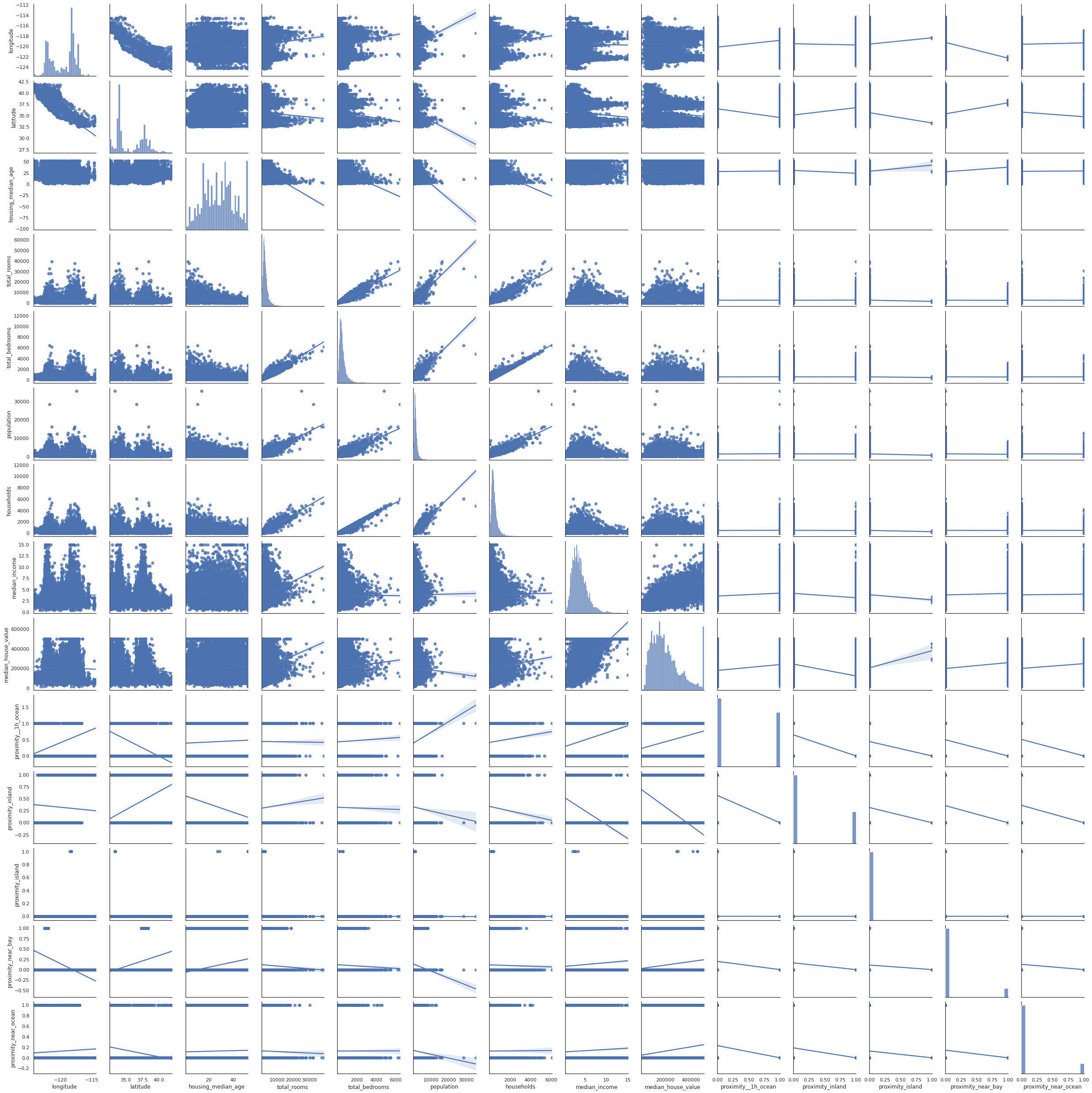### Examining subsets of variables

To work around the issue of massive and unreadable pairplots, you can split up your data frame and examine variables in batches, or you can create individual scatterplots to examine relationships of interest. For example, let’s look at `total_rooms`, `total_bedrooms`, and `households`. They are all positively correlated and could be collinear, so they may not all be required in the model.

``````plt.figure(figsize=(14,8))
sns.pairplot(df[['total_rooms','total_bedrooms','households']], kind="reg")
``````
``````<seaborn.axisgrid.PairGrid at 0x7f749461bac0>

<Figure size 1008x576 with 0 Axes>
``````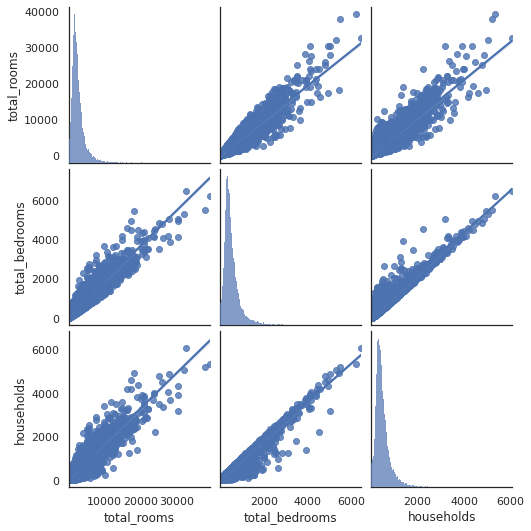### Examining pairs of variables

If you want to examine a specific pair of variables you can create a scatterplot using the `regplot()` function. This accepts an X and y argument consisting of the respective dataframe columns.

``````plt.figure(figsize=(14,8))
sns.regplot(x=df["total_rooms"], y=df["median_house_value"])
plt.title('Total rooms vs. Median house value')
``````
``````Text(0.5, 1.0, 'Total rooms vs. Median house value')
``````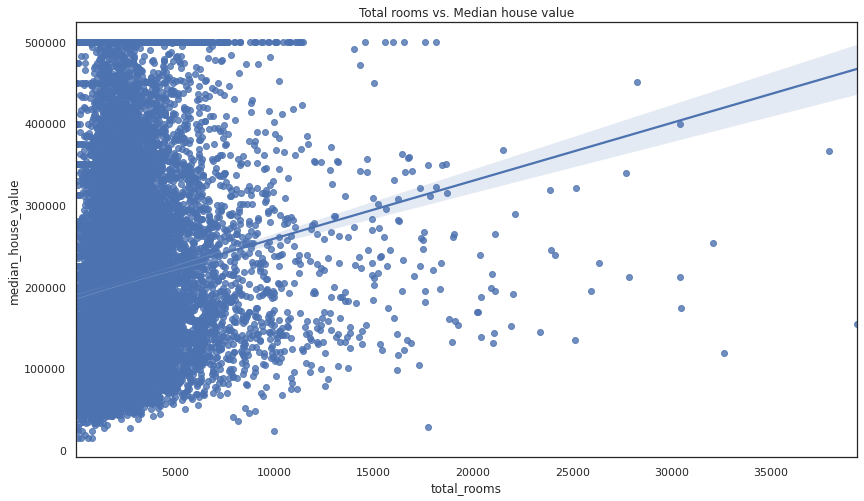Matt Clarke, Sunday, March 07, 2021

Matt Clarke Matt is an Ecommerce and Marketing Director who uses data science to help in his work. Matt has a Master's degree in Internet Retailing (plus two other Master's degrees in different fields) and specialises in the technical side of ecommerce and marketing.